# Temperature Analysis Improvement

This section explains the “resolution” and “A/D conversion,” which have a significant influence on the performance of a temperature recorder. This section also introduces measures against noise and tips for further enhancing the temperature measurement accuracy.

## Factors that determine the accuracy

There are two factors that determine the accuracy of an instrument, “resolution” and “linearity.” The combination of the two is called “accuracy” or “precision.” Let’s start with an explanation of these two factors.

### Resolution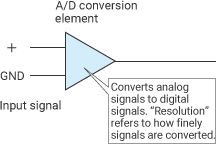Resolution refers to how finely input signals can be decomposed, which is determined by the A/D conversion element of an instrument. For example, with a resolution of 14 bits and an input range of ±5 V, the theoretical minimum reading is 0.6 mV.
±5 V/214 (16384) ≈ 0.6 mV

### Linearity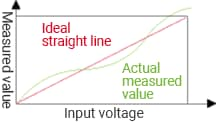In the graph on the left, input voltages are plotted on the horizontal axis whereas measured values are plotted on the vertical axis. “Linearity” indicates how accurate actual measured values are against the ideal straight line. Among (ideal values − actual measured values), the maximum amount of deviation divided by the input range is displayed as a percentage. In actual measurement, the accuracy calculated on the basis of the resolution and the linearity may not be obtained due to environmental effects (such as noise and potential difference). The two techniques listed below can be used to reduce these effects and realize accurate measurement.

• Insulation between channel grounds
• ΔΣ (delta-sigma) type A/D conversion method

## Temperature recorder performance

This section explains temperature recorder technologies that are provided to enable users to measure the temperature accurately.

### Delta-sigma A/D conversion

The delta-sigma A/D conversion method has been developed to achieve a more accurate A/D conversion than conventional, commonly used A/D conversion methods (the sequential conversion method and the flash method) by combining over-sampling and integration. This method offers extremely high performance in reproducing input signals and removing commercial power frequency noise. In particular, it is regarded to be effective in measurement with a slow sampling cycle.

#### (1) Over-sampling

“Over-sampling” refers to sampling (A/D conversion) at a higher frequency than that of input signals. This enables you to improve the reproducibility and resolution of input signals.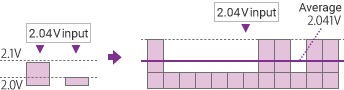When 2.04 V is input into a circuit with a resolution of 0.1 V, it is not possible to distinguish the input voltage between 2.0 V and 2.1 V. In this case, by performing over-sampling 12 times and averaging the results, you can obtain 2.041 V, which corresponds to the input value.

#### (2) Integration

Because the frequency of noise is often the same as that of the commercial power supply, simple averaging is performed for a certain period of time to reduce the effect of noise. Set and use the same integral time as that for the commercial frequency (20 ms for 50 Hz areas and 16.7 ms for 60 Hz areas).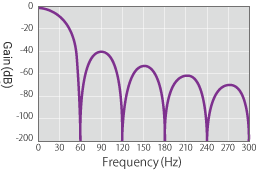The KEYENCE NR Series is equipped with an auto start at power-on function. When this function is set to ON, data collection is automatically started at power-on.

### Insulation between channel GNDs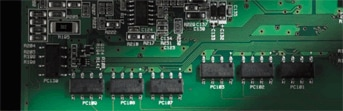This technology insulates each channel in an input circuit with a high voltage semiconductor relay to reduce the effect of common mode noise. By using this together with the delta-sigma A/D conversion method described above, you can realize accurate temperature measurement that cuts off both common and normal mode noise.

Insulation between each channel in an input circuit also enables measurement even with a potential difference between each signal GND.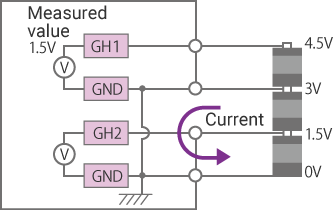If input channel GNDs are not insulated from each other and there is a potential difference between signal source GNDs, current flows between the GNDs, thereby disabling measurement.

### Heat distribution uniforming structure

When a thermocouple is used for temperature measurement, the temperature difference between the target point and the input terminal block of the temperature recorder is measured, and then the temperature of the input terminal block is added to the results. As such, errors are caused by the temperature variation between channels of the input terminal block of the temperature recorder. The heat distribution uniforming structure is a technology that uses an active heating control to reduce the temperature variation between channels. This technology has the effect of improving the measurement accuracy about twofold.

## Measures against noise

For a recorder whose GNDs in the input circuit are not insulated, caution is required for use of a grounded type thermocouple. When the measurement target, thermocouple, and GND form a circuit, noise components may enter the circuit. These noise components are called conductive noise. On the other hand, conductors such as thermocouples may often catch noise from the surroundings with the “antenna effect.” This noise is called radiation noise, which has an equivalent effect if a grounded type thermocouple is attached to a large conductor. Radiation noise, despite its simple name, has a wide variety of causes, sizes, and frequencies, each of which requires different investigation into the causes and countermeasures. However, the most common type of radiation noise is commercial power supply noise.

### Measures to prevent conductive noise from a thermocouple

• Insulate the tip of the thermocouple with tape or other means. This is a simple measure, although the responsiveness is sacrificed.
• Use a recorder in which channels are insulated.

### Measures to prevent radiation noise from a power supply

• Do not wire the power supply and measurement lines in parallel.
Of special note, be sure to wire the measurement line away from power lines.
• Electromagnetically shield the affected signal line.

### Image of delta-sigma A/D conversion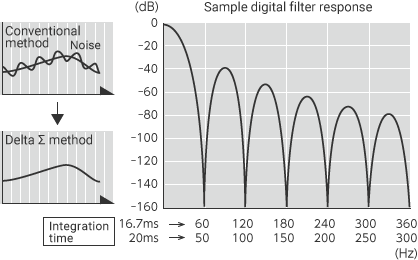INDEX